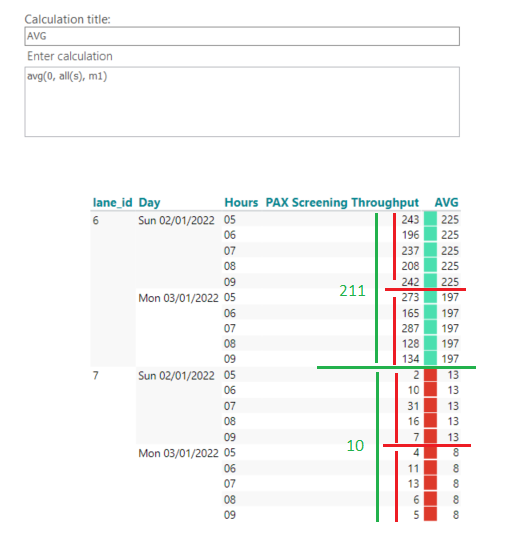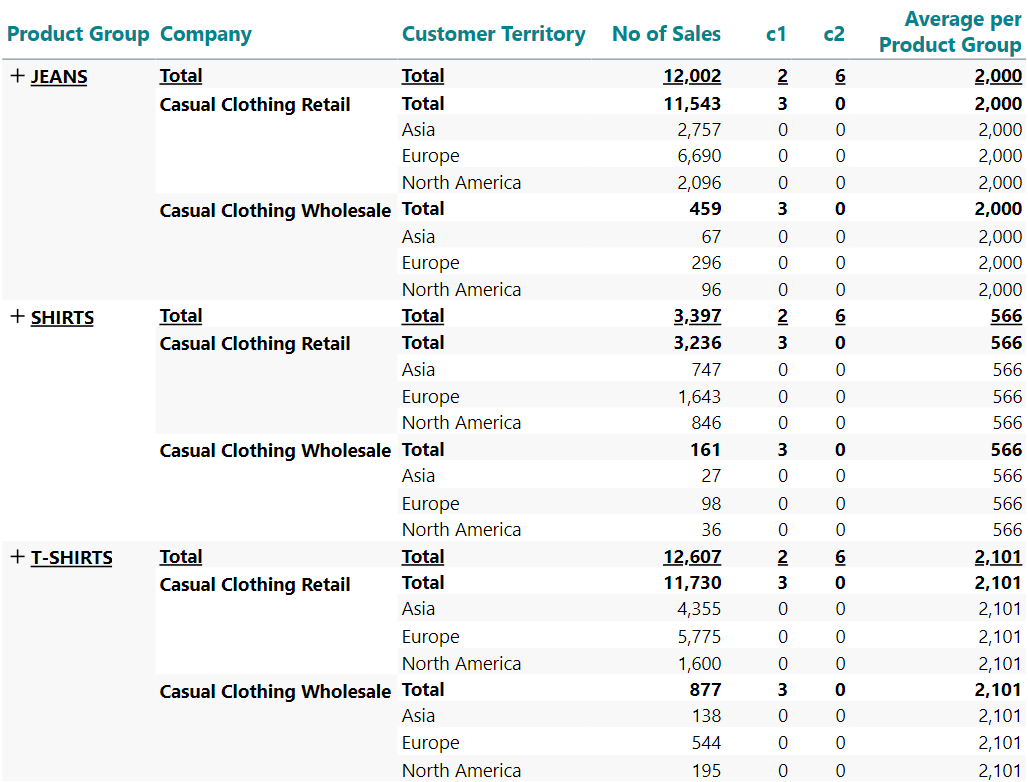# Calculate average at highest hierachy levelAs shown in the attached picture (green numbers) I'm trying to provide the average hourly m1 per lane_id (highest level in the hierarchy). With the current calculation I'm only able to avg them per day. Is there a way to combine both sibling and level modifiers to achieve this?

• Hi Joseba,

You will need to include a couple of intermediate calculations, to get to the desired result.

In my example, I also have a three-level hierarchy - like yours.No of Sales is my measure; C1 and C2 are the necessary intermediate calculations (inserted as calculated columns).

`C1: allcount(d1, all(c), m1)C2: sum(c1, all(c), m1)Average per Product Group: sum(d1, 0(l(1,0,0)), m1) / sum(c2, 0(l(1,0,0)), m1)`

For clarity, I kept the subtotals in my example. You can hide the subtotals, and the calculations will still work.

If you have a four-level hierarchy, the necessary calculation would be:

`C1: allcount(d1, all(c), m1)C2: sum(c1, all(c), m1)C3: sum(c2, all(c), m1)Average per Product Group: sum(d1, 0(l(1,0,0,0)), m1) / sum(c3, 0(l(1,0,0,0)), m1)`

Etc.

BR / Ole

• Thanks for your support Ole.

Best regards,

Joseba

• Following the case above, I'm trying to keep only the first entry of each Product Group and the corresponding Average calculated before (JEANS: 2000, SHIRTS: 566, T-SHIRTS: 2101). I hid Company, Customer Territory, No. of sales, c1 and c2. After I add a visibility agent to only show the first member of each Product Group (hiding all siblings whose position is > 1) using the formula allcount(d1, d1:0(s), m1) > 1. I also tried specifying the level in which this should be applied (l0) but I wouldn't get the desired result. Any suggestion of how can achieve this?

• Hi Joseba

I think the problem is that TARGIT counts on all levels, which means that the count also is done on subtotal level.

To ensure that the visibility agent only evaluates one the most granular level, you need to check if you are evaluating a subtotal.

What is special about subtotals is that the have "children" - meaning the individual values the adds up to the subtotal.

The modifier for children is (c).

Your visibilty agent shold have this syntax:

allcount(d1, d1:0(s), m1) > 1 and allcount(d1, all(c), m1) = 0

The second part of the syntax (after the and) counts all the children for a certain row. If this amounts to zero children, then we are looking at the most granular level of the table at hand.

Hope it makes sense.

• That makes totally sense Niels. Thanks for your support!